GeeksforGeeks App
Open AppBrowser
Continue

## Related Articles

• Write an Interview Experience
• Mathematical Algorithms

# Find the Factorial of a large number

Find the factorial of a large number.

## What is Factorial of a number?

Factorial of a non-negative integer, is the multiplication of all integers smaller than or equal to n.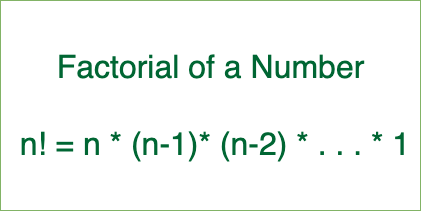Factorial of a number

Examples:

Input: 100
Output: 933262154439441526816992388562667004-
907159682643816214685929638952175999-
932299156089414639761565182862536979-
208272237582511852109168640000000000-
00000000000000

Input: 50
Output: 3041409320171337804361260816606476884-
4377641568960512000000000000

We have discussed a simple program for factorial.

## Why conventional way of computing factorial fails for large numbers?

A factorial of 100 has 158 digits. It is not possible to store these many digits even if we use long int.

The idea is to use basic mathematics for multiplication.

Illustration:

Example to show working of multiply(res[], x)

• A number 5189 is stored in res[] as following: res[] = {9, 8, 1, 5}
• let x = 10
Initialize carry = 0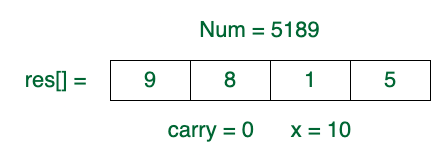• At i = 0, prod = res*x + carry = 9*10 + 0 = 90.
res = 0, carry = 9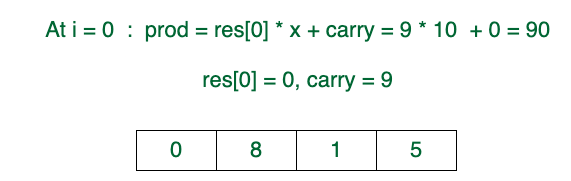• At i = 1, prod = res*x + carry = 8*10 + 9 = 89
res = 9, carry = 8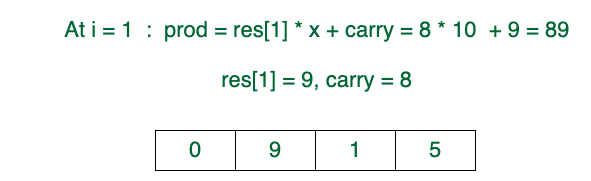• At i = 2, prod = res*x + carry = 1*10 + 8 = 18
res = 8, carry = 1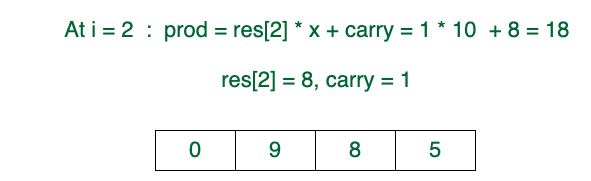• At i = 3, prod = res*x + carry = 5*10 + 1 = 51
res = 1, carry = 5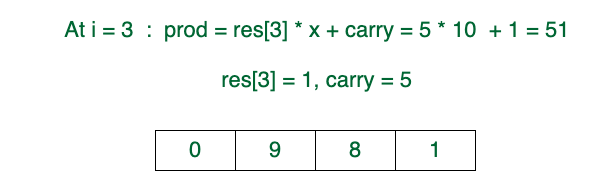• res = carry = 5
res[] = {0, 9, 8, 1, 5}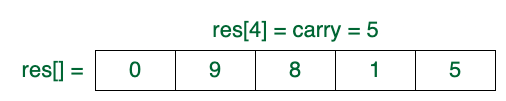Follow the steps below to solve the given problem:

• Create an array res[] of MAX size where MAX is a number of maximum digits in output.
• Initialize value stored in res[] as 1 and initialize res_size (size of ‘res[]’) as 1.
• Multiply x with res[] and update res[] and res_size to store the multiplication result for all the numbers from x = 2 to n.
• To multiply a number x with the number stored in res[], one by one multiply x with every digit of res[].
• To implement multiply function perform the following steps:
• Initialize carry as 0.
• Do following for i = 0 to res_size – 1
• Find value of res[i] * x + carry. Let this value be prod.
• Update res[i] by storing the last digit of prod in it.
• Update carry by storing the remaining digits in carry.
• Put all digits of carry in res[] and increase res_size by the number of digits in carry.

Below is the implementation of the above algorithm.

NOTE: In the below implementation, the maximum digits in the output are assumed as 500. To find a factorial of a much larger number ( > 254), increase the size of an array or increase the value of MAX. This can also be solved using Linked List instead of using res[] array which will not waste extra space.

## C++

 `// C++ program to compute factorial of big numbers``#include ``using` `namespace` `std;` `// Maximum number of digits in output``#define MAX 500` `int` `multiply(``int` `x, ``int` `res[], ``int` `res_size);` `// This function finds factorial of large numbers``// and prints them``void` `factorial(``int` `n)``{``    ``int` `res[MAX];` `    ``// Initialize result``    ``res = 1;``    ``int` `res_size = 1;` `    ``// Apply simple factorial formula n! = 1 * 2 * 3``    ``// * 4...*n``    ``for` `(``int` `x = 2; x <= n; x++)``        ``res_size = multiply(x, res, res_size);` `    ``cout << ``"Factorial of given number is \n"``;``    ``for` `(``int` `i = res_size - 1; i >= 0; i--)``        ``cout << res[i];``}` `// This function multiplies x with the number``// represented by res[].``// res_size is size of res[] or number of digits in the``// number represented by res[]. This function uses simple``// school mathematics for multiplication.``// This function returns the``// new value of res_size``int` `multiply(``int` `x, ``int` `res[], ``int` `res_size)``{``    ``int` `carry = 0; ``// Initialize carry` `    ``// One by one multiply n with individual digits of res[]``    ``for` `(``int` `i = 0; i < res_size; i++) {``        ``int` `prod = res[i] * x + carry;` `        ``// Store last digit of 'prod' in res[]``        ``res[i] = prod % 10;` `        ``// Put rest in carry``        ``carry = prod / 10;``    ``}` `    ``// Put carry in res and increase result size``    ``while` `(carry) {``        ``res[res_size] = carry % 10;``        ``carry = carry / 10;``        ``res_size++;``    ``}``    ``return` `res_size;``}` `// Driver program``int` `main()``{``    ``factorial(100);``    ``return` `0;``}`

## Java

 `// JAVA program to compute factorial``// of big numbers``class` `GFG {` `    ``// This function finds factorial of``    ``// large numbers and prints them``    ``static` `void` `factorial(``int` `n)``    ``{``        ``int` `res[] = ``new` `int``[``500``];` `        ``// Initialize result``        ``res[``0``] = ``1``;``        ``int` `res_size = ``1``;` `        ``// Apply simple factorial formula``        ``// n! = 1 * 2 * 3 * 4...*n``        ``for` `(``int` `x = ``2``; x <= n; x++)``            ``res_size = multiply(x, res, res_size);` `        ``System.out.println(``"Factorial of given number is "``);``        ``for` `(``int` `i = res_size - ``1``; i >= ``0``; i--)``            ``System.out.print(res[i]);``    ``}` `    ``// This function multiplies x with the number``    ``// represented by res[]. res_size is size of res[] or``    ``// number of digits in the number represented by res[].``    ``// This function uses simple school mathematics for``    ``// multiplication. This function may value of res_size``    ``// and returns the new value of res_size``    ``static` `int` `multiply(``int` `x, ``int` `res[], ``int` `res_size)``    ``{``        ``int` `carry = ``0``; ``// Initialize carry` `        ``// One by one multiply n with individual``        ``// digits of res[]``        ``for` `(``int` `i = ``0``; i < res_size; i++) {``            ``int` `prod = res[i] * x + carry;``            ``res[i] = prod % ``10``; ``// Store last digit of``                                ``// 'prod' in res[]``            ``carry = prod / ``10``; ``// Put rest in carry``        ``}` `        ``// Put carry in res and increase result size``        ``while` `(carry != ``0``) {``            ``res[res_size] = carry % ``10``;``            ``carry = carry / ``10``;``            ``res_size++;``        ``}``        ``return` `res_size;``    ``}` `    ``// Driver program``    ``public` `static` `void` `main(String args[])``    ``{``        ``factorial(``100``);``    ``}``}``// This code is contributed by Nikita Tiwari`

## Python3

 `# Python program to compute factorial``# of big numbers` `import` `sys` `# This function finds factorial of large``# numbers and prints them`  `def` `factorial(n):``    ``res ``=` `[``None``]``*``500``    ``# Initialize result``    ``res[``0``] ``=` `1``    ``res_size ``=` `1` `    ``# Apply simple factorial formula``    ``# n! = 1 * 2 * 3 * 4...*n``    ``x ``=` `2``    ``while` `x <``=` `n:``        ``res_size ``=` `multiply(x, res, res_size)``        ``x ``=` `x ``+` `1` `    ``print``(``"Factorial of given number is"``)``    ``i ``=` `res_size``-``1``    ``while` `i >``=` `0``:``        ``sys.stdout.write(``str``(res[i]))``        ``sys.stdout.flush()``        ``i ``=` `i ``-` `1`  `# This function multiplies x with the number``# represented by res[]. res_size is size of res[]``# or number of digits in the number represented``# by res[]. This function uses simple school``# mathematics for multiplication. This function``# may value of res_size and returns the new value``# of res_size``def` `multiply(x, res, res_size):` `    ``carry ``=` `0`  `# Initialize carry` `    ``# One by one multiply n with individual``    ``# digits of res[]``    ``i ``=` `0``    ``while` `i < res_size:``        ``prod ``=` `res[i] ``*` `x ``+` `carry``        ``res[i] ``=` `prod ``%` `10`  `# Store last digit of``        ``# 'prod' in res[]``        ``# make sure floor division is used``        ``carry ``=` `prod``/``/``10`  `# Put rest in carry``        ``i ``=` `i ``+` `1` `    ``# Put carry in res and increase result size``    ``while` `(carry):``        ``res[res_size] ``=` `carry ``%` `10``        ``# make sure floor division is used``        ``# to avoid floating value``        ``carry ``=` `carry ``/``/` `10``        ``res_size ``=` `res_size ``+` `1` `    ``return` `res_size`  `# Driver program``factorial(``100``)` `# This code is contributed by Nikita Tiwari.`

## C#

 `// C# program to compute``// factorial of big numbers``using` `System;` `class` `GFG {` `    ``// This function finds factorial``    ``// of large numbers and prints them``    ``static` `void` `factorial(``int` `n)``    ``{``        ``int``[] res = ``new` `int``;` `        ``// Initialize result``        ``res = 1;``        ``int` `res_size = 1;` `        ``// Apply simple factorial formula``        ``// n! = 1 * 2 * 3 * 4...*n``        ``for` `(``int` `x = 2; x <= n; x++)``            ``res_size = multiply(x, res, res_size);` `        ``Console.WriteLine(``"Factorial of "``                          ``+ ``"given number is "``);``        ``for` `(``int` `i = res_size - 1; i >= 0; i--)``            ``Console.Write(res[i]);``    ``}` `    ``// This function multiplies x``    ``// with the number represented``    ``// by res[]. res_size is size``    ``// of res[] or number of digits``    ``// in the number represented by``    ``// res[]. This function uses``    ``// simple school mathematics for``    ``// multiplication. This function``    ``// may value of res_size and``    ``// returns the new value of res_size``    ``static` `int` `multiply(``int` `x, ``int``[] res, ``int` `res_size)``    ``{``        ``int` `carry = 0; ``// Initialize carry` `        ``// One by one multiply n with``        ``// individual digits of res[]``        ``for` `(``int` `i = 0; i < res_size; i++) {``            ``int` `prod = res[i] * x + carry;``            ``res[i] = prod % 10; ``// Store last digit of``                                ``// 'prod' in res[]``            ``carry = prod / 10; ``// Put rest in carry``        ``}` `        ``// Put carry in res and``        ``// increase result size``        ``while` `(carry != 0) {``            ``res[res_size] = carry % 10;``            ``carry = carry / 10;``            ``res_size++;``        ``}``        ``return` `res_size;``    ``}` `    ``// Driver Code``    ``static` `public` `void` `Main() { factorial(100); }``}` `// This code is contributed by ajit`

## PHP

 `= 0; ``\$i``--)``        ``echo` `\$res``[``\$i``];``}` `// This function multiplies x with the number``// represented by res[].``// res_size is size of res[] or number of``// digits in the number represented by res[].``// This function uses simple school mathematics``// for multiplication. This function may value ``// of res_size and returns the new value of res_size``function` `multiply(``\$x``, &``\$res``, ``\$res_size``)``{``    ``\$carry` `= 0; ``// Initialize carry` `    ``// One by one multiply n with individual``    ``// digits of res[]``    ``for` `(``\$i` `= 0; ``\$i` `< ``\$res_size``; ``\$i``++)``    ``{``        ``\$prod` `= ``\$res``[``\$i``] * ``\$x` `+ ``\$carry``;` `        ``// Store last digit of 'prod' in res[]``        ``\$res``[``\$i``] = ``\$prod` `% 10;` `        ``// Put rest in carry``        ``\$carry` `= (int)(``\$prod` `/ 10);``    ``}` `    ``// Put carry in res and increase``    ``// result size``    ``while` `(``\$carry``)``    ``{``        ``\$res``[``\$res_size``] = ``\$carry` `% 10;``        ``\$carry` `= (int)(``\$carry` `/ 10);``        ``\$res_size``++;``    ``}``    ``return` `\$res_size``;``}` `// Driver Code``factorial(100);``    ` `// This code is contributed by chandan_jnu``?>`

## Javascript

 ``

Output

```Factorial of given number is
93326215443944152681699238856266700490715968264381621468592963895217599993229915608941463976156518286253697920827223758251185210916864000000000000000000000000```

Time Complexity: O(N log (N!)), where O(N) is for loop and O(log N!) is for nested while loop
Auxiliary Space: O(max(digits in factorial))

## Find the Factorial of a large number using Basic BigInteger

This problem can be solved using the below idea:

Big Integer can also be used to calculate the factorial of large numbers.

Illustration:

N = 5

ans = 1

At i = 2: ans = ans x i = 1 x 2 = 2
At i = 3: ans = ans x i = 2 x 3 = 6
At i = 4: ans = ans x i = 6 x 4 = 24
At i = 5: ans = ans x i = 24 x 5 = 120

Hence factorial of N is 120

Follow the steps below to solve the given problem:

• Declare a BigInteger f with 1 and perform the conventional way of calculating factorial
• Traverse a loop from x = 2 to N and multiply x with f and store the resultant value in f

Below is the implementation of the above idea :

## C++

 `// C++ program to find large``// factorials using BigInteger``#include ``using` `namespace` `std;` `#define ull unsigned long long` `// Returns Factorial of N``ull factorial(``int` `N)``{` `    ``// Initialize result``    ``ull f = 1; ``// Or BigInt 1` `    ``// Multiply f with 2, 3, ...N``    ``for` `(ull i = 2; i <= N; i++)``        ``f *= i;` `    ``return` `f;``}` `// Driver method``int` `main()``{``    ``int` `N = 20;``    ``cout << factorial(N) << endl;``}` `// This code is contributed by phasing17`

## Java

 `// Java program to find large``// factorials using BigInteger``import` `java.math.BigInteger;``import` `java.util.Scanner;` `public` `class` `Example {` `    ``// Returns Factorial of N``    ``static` `BigInteger factorial(``int` `N)``    ``{``        ``// Initialize result``        ``BigInteger f``            ``= ``new` `BigInteger(``"1"``); ``// Or BigInteger.ONE` `        ``// Multiply f with 2, 3, ...N``        ``for` `(``int` `i = ``2``; i <= N; i++)``            ``f = f.multiply(BigInteger.valueOf(i));` `        ``return` `f;``    ``}` `    ``// Driver method``    ``public` `static` `void` `main(String args[]) ``throws` `Exception``    ``{``        ``int` `N = ``20``;``        ``System.out.println(factorial(N));``    ``}``}`

## Python3

 `# Python3 program to find large``# factorials` `# Returns Factorial of N``def` `factorial(N):` `    ``# Initialize result``    ``f ``=`  `1` `    ` `    ``# Multiply f with 2, 3, ...N``    ``for` `i ``in` `range``(``2``, N ``+` `1``):``        ``f ``*``=` `i``    ` `    ``return` `f;`  `# Driver method``N ``=` `20``;``print``(factorial(N));`  `# This code is contributed by phasing17`

## C#

 `// C# program to find large``// factorials using BigInteger``using` `System;``using` `System.Collections.Generic;``using` `System.Numerics;` `public` `class` `Example {` `  ``// Returns Factorial of N``  ``static` `BigInteger factorial(``int` `N)``  ``{` `    ``// Initialize result``    ``BigInteger f``      ``= ``new` `BigInteger(1); ``// Or BigInteger.ONE` `    ``// Multiply f with 2, 3, ...N``    ``for` `(``int` `i = 2; i <= N; i++)``      ``f = BigInteger.Multiply(f, ``new` `BigInteger(i));` `    ``return` `f;``  ``}` `  ``// Driver method``  ``public` `static` `void` `Main(``string``[] args)``  ``{``    ``int` `N = 20;``    ``Console.WriteLine(factorial(N));``  ``}``}` `// This code is contributed by phasing17`

## Javascript

 `// JavaScript program to find large``// factorials using BigInteger` `// Returns Factorial of N``function` `factorial(N)``{` `// Initialize result``let f =  BigInt(1); ``// Or BigInt 1` `// Multiply f with 2, 3, ...N``for` `(``var` `i = 2; i <= N; i++)``    ``f *= BigInt(i);` `return` `f;``}` `// Driver method``let N = 20;``console.log(factorial(N));` `// This code is contributed by phasing17`

Output

`2432902008176640000`

Time Complexity: O(n)
Auxiliary Space: O(1)

### Method 3:

1. Define a function factorial(n) that takes an integer n as input.
2. Check if n is 0 or 1. If it is, return 1.
3. If n is greater than 1, call the function recursively with input n-1 and store the result in a variable called sub_result.
4. Multiply sub_result with n and return the product as the final result.

## C++

 `#include ``#include ` `using` `namespace` `std;` `vector<``int``> multiply(vector<``int``>& digits, ``int` `factor) {``    ``int` `carry = 0;``    ``for` `(``int` `i = 0; i < digits.size(); i++) {``        ``int` `prod = digits[i] * factor + carry;``        ``digits[i] = prod % 10;``        ``carry = prod / 10;``    ``}``    ``while` `(carry) {``        ``digits.push_back(carry % 10);``        ``carry /= 10;``    ``}``    ``return` `digits;``}` `void` `print(vector<``int``>& digits) {``    ``for` `(``int` `i = digits.size() - 1; i >= 0; i--) {``        ``cout << digits[i];``    ``}``}` `int` `main() {``    ``int` `n = 100;``    ``vector<``int``> digits;``    ``digits.push_back(1);``    ``for` `(``int` `i = 2; i <= n; i++) {``        ``digits = multiply(digits, i);``    ``}``    ``print(digits);``    ``return` `0;``}`

## Java

 `import` `java.math.BigInteger;` `public` `class` `Main {``    ``public` `static` `void` `main(String[] args) {``        ``int` `n = ``100``;``        ``BigInteger result = BigInteger.valueOf(``1``);``        ``for` `(``int` `i = ``1``; i <= n; i++) {``            ``result = result.multiply(BigInteger.valueOf(i));``        ``}``        ``System.out.println(result);``    ``}``}`

## Python3

 `import` `math` `n ``=` `100``result ``=` `math.factorial(n)``print``(result)`

## Javascript

 `function` `factorial(n) {``  ` `  ``let result = BigInt(1);``  ` `  ``for` `(let i = 2; i <= n; i++) {``    ``result *= BigInt(i);``  ``}``  ` `  ``return` `result;``}` `// Set the value of n``const n = 100;` `// Calculate the factorial of n using the math library``const result = factorial(n);` `// Print the result``console.log(result);`

## C#

 `// C# program for the above approach``using` `System;``using` `System.Collections.Generic;` `class` `MainClass {` `    ``// Function to multiply the two integers``    ``static` `List<``int``> Multiply(List<``int``> digits, ``int` `factor)``    ``{``        ``int` `carry = 0;``        ``for` `(``int` `i = 0; i < digits.Count; i++) {``            ``int` `prod = digits[i] * factor + carry;``            ``digits[i] = prod % 10;``            ``carry = prod / 10;``        ``}``        ``while` `(carry > 0) {``            ``digits.Add(carry % 10);``            ``carry /= 10;``        ``}` `        ``// Return the digits``        ``return` `digits;``    ``}` `    ``// Print the list``    ``static` `void` `Print(List<``int``> digits)``    ``{``        ``for` `(``int` `i = digits.Count - 1; i >= 0; i--) {``            ``Console.Write(digits[i]);``        ``}``    ``}` `    ``// Driver Code``    ``static` `void` `Main()``    ``{``        ``int` `n = 100;``        ``List<``int``> digits = ``new` `List<``int``>();``        ``digits.Add(1);``        ``for` `(``int` `i = 2; i <= n; i++) {``            ``digits = Multiply(digits, i);``        ``}``        ``Print(digits);``    ``}``}`

Output

`93326215443944152681699238856266700490715968264381621468592963895217599993229915608941463976156518286253697920827223758251185210916864000000000000000000000000`

Time Complexity: O(n)

The time complexity of the code is O(n), where n is the value of the input parameter. This is because the math.factorial() function internally uses a loop to calculate the product of all integers from 1 to n, and the loop iterates n times. Therefore, the time taken by the function is proportional to n.
Auxiliary Space: O(1)

The auxiliary space complexity of the code is O(1), because the code uses only a constant amount of additional memory to store the result of the factorial calculation. Specifically, the memory required to store the result is proportional to the number of digits in the result, which is roughly log10(n!), or O(log n), but this is negligible compared to the input size for large values of n.

My Personal Notes arrow_drop_up